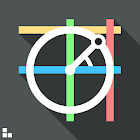Trigonometry. Unit circle.

All Android applications categories

All Android games categories# Trigonometry. Unit circle.

by: 3.5K 9.2

9.2 Users
rating

## Screenshots

Description

Education for all. Geometry. Trigonometry. Unit circle.
Recommended for pupils and students.

Visual understanding and calculating sine, cosine, tangent, cotangent, secant and cosecant function, degrees and radians.
Description of functions.
Table of values.

Trigonometric formulas and identities:
symmetry, shifts, periodicity,
basic identities,
sum and difference of the angles,
double angle,
triple angle
half-angle,
sum and difference functions
multiplication of functions,
lowering the degree formula,
derivatives and integrals.

Move point to define angle and functions.
Tap circle center to determine the exact angle you want.
Use options to show what you want.

Find mistake or did not find what you were looking. - please send us e-mail.

Tags: tcircle

## Users review

from 3.530 reviews

"Awesome"

9.2